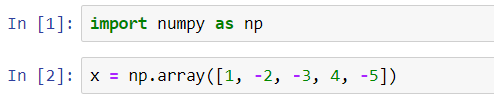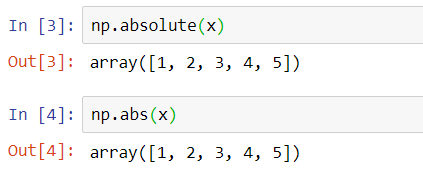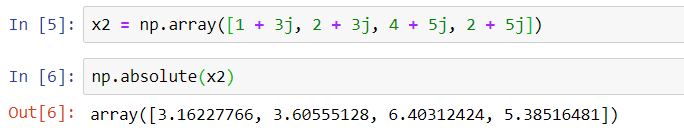The absolute value function in the NumPy array calculates the absolute value of each and every element in the array.For calculating the absolute value, NumPy gives us the np.absolute(x) & np.abs(x) function.We can also calculate the absolute values of complex numbers in NumPy.